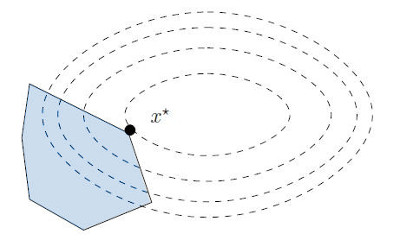Quadratic programs are a particular kind of mathematical optimization problems that can be applied to solve a variety of problems, for instance: in statistics for curve fitting, in machine learning to compute support vector machines (SVMs), in robotics to solve inverse kinematics, etc. They are the first step beyond linear programming (LP) in convex optimization.

## Standard form

A quadratic program (QP) is written in standard form as:

\begin{equation*} \begin{array}{rl} \mathrm{minimize} & (1/2) x^T P x + q^T x \\ \mathrm{subject\ to} & G x \leq h \\ & A x = b \end{array} \end{equation*}

Here, $$x$$ is the vector of optimization variables $$x_1, \ldots, x_n$$. The matrix $$P$$ and vector $$q$$ are used to define any quadratic objective function on these variables, while the matrix-vector couples $$(G, h)$$ and $$(A, b)$$ are used to define inequality and equality constraints, respectively. Vector inequalities apply coordinate by coordinate.

This mathematical construction means the following: a QP finds the minimum of a quadratic function over a linear set:In the 2D illustration above, the level sets of the quadratic function are represented by ellipses, and the linear set is the blue polygon. Since the global optimal of the objective function is outside of the polygon, the solution $$x^*$$ of the QP lies at the boundary of the linear set. The set of linear constraints that are saturated at $$x^*$$ is called the active set, but that's a story for another post...

Back to the standard form, notice that there is no constant term in the objective function. Indeed, it would have no effect on the result of the optimization, which is the location of the solution $$x^*$$. Expressions like $$\| A x - b \|^2$$ are written in standard form as $$x^T (A^T A) x - 2 (A^T b)^T x$$.

The standard form also assumes without loss of generality that the matrix $$P$$ is symmetric. Any matrix $$M$$ can be decomposed as sum of its symmetric part $$M^+$$ and antisymmetric part $$M^-$$, and the latter yields zero in $$x^T M^- x$$. Note that some QP solvers assume that you feed them a symmetric cost matrix: they won't check this, and will return wrong results if you don't.

## Small example

Below I assume that you have access to a solve_qp function that solves quadratic programs in standard form. For instance, the qpsolvers module provides one directly if you are using Python. Let us then see how to solve the following QP:

\begin{align*} \begin{array}{rl} \mathrm{minimize} & \left\| \left[\begin{array}{ccc} 1 & 2 & 0 \\ -8 & 3 & 2 \\ 0 & 1 & 1 \end{array}\right] \left[\begin{array}{c} x_1 \\ x_2 \\ x_3\end{array}\right] - \left[\begin{array}{c} 3 \\ 2 \\ 3\end{array}\right] \right\|^2 \\ \mathrm{subject\ to} & \left[\begin{array}{ccc} 1 & 2 & 1 \\ 2 & 0 & 1 \\ -1 & 2 & -1 \end{array}\right] \left[\begin{array}{c} x_1 \\ x_2 \\ x_3\end{array}\right] \leq \left[\begin{array}{c} 3 \\ 2 \\ -2 \end{array} \right] \end{array} \end{align*}

First, we write our QP matrices in proper format:

M = array([[1., 2., 0.], [-8., 3., 2.], [0., 1., 1.]])
P = dot(M.T, M)
q = dot(array([3., 2., 3.]), M).reshape((3,))
G = array([[1., 2., 1.], [2., 0., 1.], [-1., 2., -1.]])
h = array([3., 2., -2.]).reshape((3,))


Finally, we compute the solution using the function above:

In : solve_qp(P, q, G, h)
Out: array([-0.49025721, -1.57755261, -0.66484801])


## To go further

The qpsolvers Python module implements the solve_qp function for a variety of QP solvers. You can also check out this more practical blog post on Quadratic Programming in Python.

Pages of this website are under the CC-BY 4.0 license.